Reconstruction of the 2016 Chilcomb Down formation 1.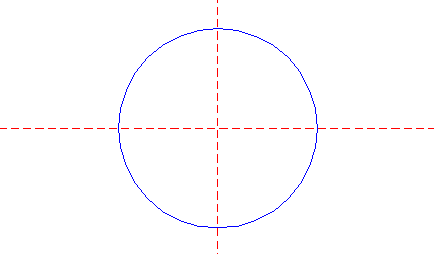Draw a circle. Draw and extend the horizontal and vertical centerlines. 2.Copy circle 1 to its righthand intersection with the horizontal centerline. 3.Construct a circle concentric to circle 1, tangent to circle 2 at the righthand side. 4.Construct the inscribed hexagon (regular 6-sided polygon) of circle 3, pointing to the right. 5.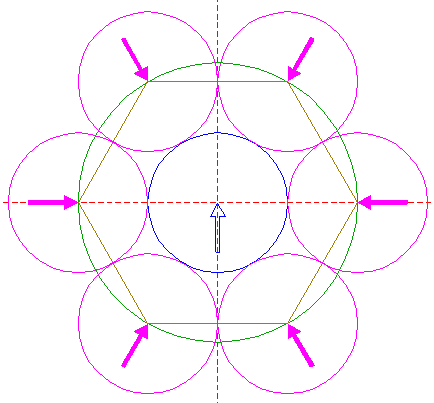Copy circle 1 six times, to the angular points of hexagon 4. 6.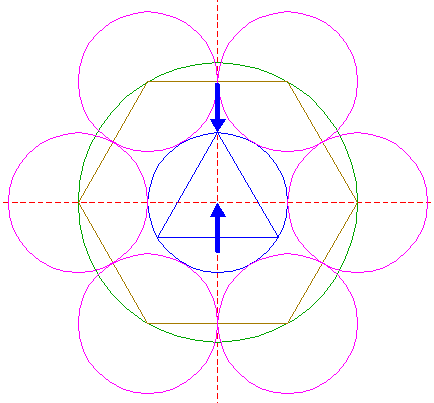Construct the inscribed equilateral triangle of circle 1, pointing up. 7.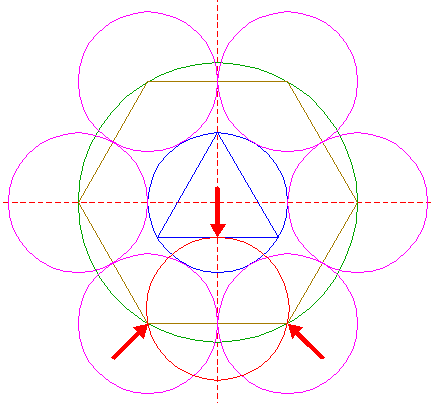Construct a circle, passing through the lower two angular points of hexagon 4, and tangent to the lower side of triangle 6. 8.Construct a circle concentric to circle 1, passing through the center of circle 7. 9.Construct the inscribed hexagon of circle 8, pointing down. 10.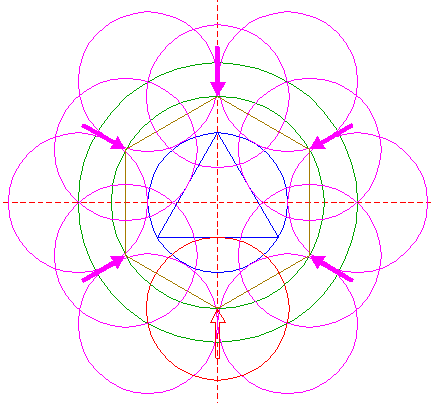Copy circle 7 five times, to the other angular points of hexagon 9. 11.Construct the inscribed circle of triangle 6. 12.Construct the inscribed hexagon of circle 11, pointing down. 13.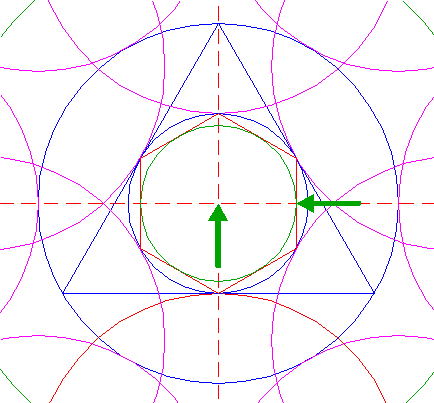Construct the inscribed circle of hexagon 12. 14.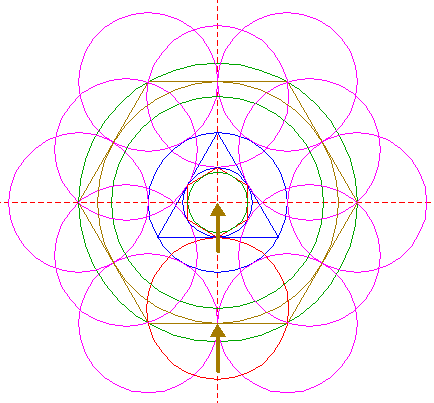Construct the inscribed circle of hexagon 4. 15.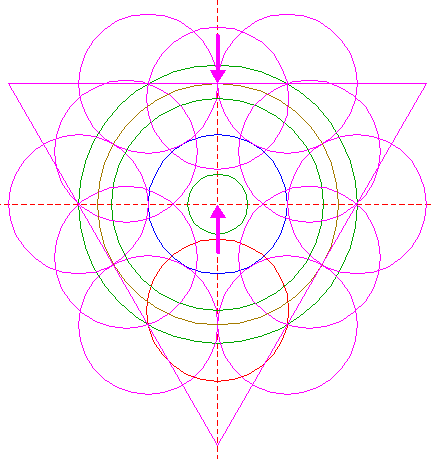Construct the circumscribed equilateral triangle of circle 14, pointing down. 16.Copy circle 13 to the lower angular point of triangle 15. 17.Construct a circle concentric to circle 1, tangent to circle 16 at the lower side. 18.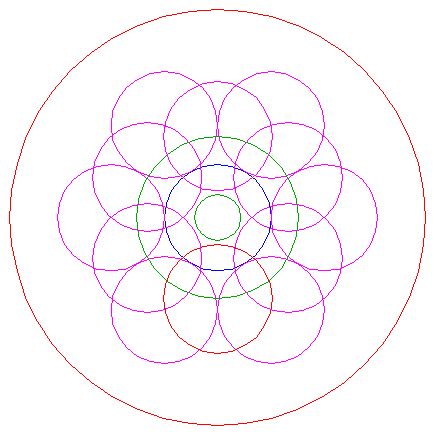Circles 1, 5, 7, 8, 10, 13, and 17, are used for the final reconstruction. 19.Remove all parts not visible within the formation itself. 20.Colour all areas corresponding to standing... 21.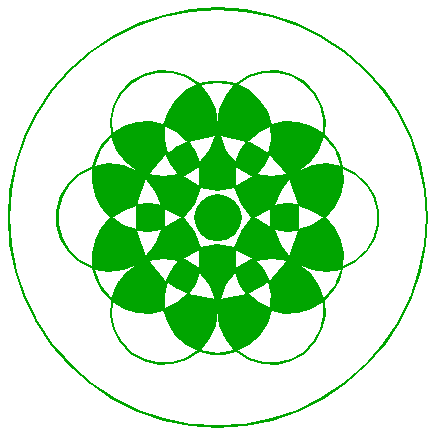...or to flattened crop, and finish the reconstruction of the 2016 Chilcomb Down formation. 22.The final result, matched with the aerial image.
 Copyright © 2016, Zef Damen, The NetherlandsMark Fussell & Stuart Dike